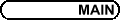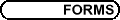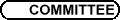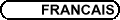Dynamical Systems and Applications / Systèmes dynamiques

WILLIAM BASENER, Rochester Institute of Technology
Booming and Crashing Population Dynamics and Easter Island

We present a modified predator-prey ODE modeling an isolated population and its resources. Numerical results using appropriate parameters match archeological data for the human population on Easter Island. This is in contrast to standard models, which do not predict the booming and crashing of the Easter Island population. A complete qualitative analysis of the model is presented, including a 2D degenerate Hopf bifurcation and a Lyapanov function. Other applications of this model are also considered.

LORA BILLINGS, Montclair State University
Stochastic Epidemic Outbreaks: Why Epidemics Are Like Lasers

Many diseases, such as childhood diseases, dengue fever, and West Nile virus, appear to oscillate randomly as a function of seasonal environmental or social changes. Such oscillations appear to have a chaotic bursting character, although it is still uncertain how much is due to random fluctuations. Such bursting in the presence of noise is also observed in driven lasers. In this talk, I will show how noise can excite random outbreaks in simple models of seasonally driven outbreaks, as well as lasers. The models for both population dynamics will be shown to share the same class of underlying topology, which plays a major role in the cause of observed stochastic bursting.

STEFANELLA BOATTO, Dept. of Mathematics and Statistics, McMaster University, 1280 Main Street West, Hamilton, Ontario L8S 4K1
Periodic solutions of the Euler's equation on a sphere

Classes of steady and periodic solutions are investigated for the incompressible Euler equation on a sphere. Of particular interest is the stability of such solutions. The study makes use of an infinite dimensional Hamiltonian formulation of the vorticity equation when the rotation of a planet is taken into account.

ERIK BOLLT, Clarkson University, Potsdam, NY 13699-5815
Mapping Transport Activity in Stochastic Dynamics, Directly from The Transfer Operator and Learning Noise Induced Multistability

Associated with a dynamical system, which evolves single initial conditions, the Frobenius-Perron operator evolves ensemble densities of initial conditions. We will present our new applications of this global and statistical point of view: Well-known models have been found to exhibit new and interesting dynamics under the addition of stochastic perturbations. Generalizing the Frobenius-Perron operator to stochastic dynamical systems, we develop new tools designed to predict the effects of noise and to pinpoint stochastic transport regions in phase space in the absence of global manifold information.

BERNARD BROOKS, RIT
Discrete Population Dynamic of Easter Island

The continuous population dynamic of Easter Island has been investigated in order to try and explain the unusual historic population trends noted on the island. Archaeological evidence suggests that the island's population boomed and then, shortly before the arrival of Europeans, crashed almost to zero. The continuous model yielded very interesting results and demonstrated that there did exist a tipping point where the inhabitants could harvest too much of their resources resulting in the observed data.

This talk will focus on the analysis of the discrete version of that model. The 2-dimensional first order discrete dynamic contains the familiar logistic map to model the human population. What is not so familiar is that the resources that the population's carrying capacity depends on will also be governed by logistic growth. This modification to the well-studied logistic growth concept provides a much more realistic model of an isolated population feeding on biological resources. In addition to that added realism, the dynamic is mathematically rich hinting at the possibility of chaos.

LEO BUTLER, Queen's University, Kingston, ON K7L 3N6
Invariant fibrations of geodesic flows

A flow ft : M ® M is weakly integrable if there is an open dense set L Ì M that is fibred by invariant tori. If G = M-L is a tamely embedded polyhedron and the invariant tori are k-dimensional, then ft is k-semisimple.

Theorem: Let (S, g) be a compact riemannian 3-manifold. If its geodesic flow is 3-semisimple, then p1(S) is almost 2-step polycyclic.

The proof uses an argument due to Kozlov and Taimanov. The Evans-Moser classification theorem and work by Bolsinov and Taimanov implies a partial converse.

HAMIDEH D. HAMEDANI, Department of Statistics, Faculty of Mathematical Sciences, Shahid Beheshti University, Tehran, Iran
Stopped Semimonotone Nonlinear Stochastic Integral Equations with Martingale Noise

The theory of stochastic differential equations in Hilbert and Banach spaces have a wide range of applications in stochastic modeling to describe the dynamic of random phenomena studied in science and engineering. In this context, there are many initial value problems in which time is stopped by a given stopping time. For instance, they may occur for parabolic and hyperbolic type PDEs with a stopping condition at the boundary of the region, or for stochastic systems in bounded regions with absorption at the boundary. Hence an existence-uniqueness result plays an important role in this direction.

Consider the following semimonotone nonlinear integral equation:

 Xt = U(t,0) X0 + óõ t 0 U(t,s) F(s,Xs)  ds + óõ (0,t] U(t,s)G(s-,Xs-)  dMs + Vt     t £ t,
(1)
where

(a) U(t,s) is a contraction-type evolution operator generated by A(.).
(b) M is a K-valued cadlag square integrable martingale with arbitrary jump sizes, G Î L2 (K; H; P, M) where K and H are real separable Hilbert spaces, and P is the s-algebra of predictable sets. Ft(.) = F(t,w,.) : H ® H is demicontinuous, semimonotone and Gt(.) = G(t,w,.) : H ® L2 (K; H; P, M) is Lipschitz. Suppose that a linear growth condition as well as appropriate measurability assumptions hold for both F and G.
(c) Vt is an H-valued cadlag adapted process and X0 is a random variable.

Using Picard iterated method, we prove that the integral equation (1) has a unique strong measurable solution. We also show the continuity of the solution with respect to the initial condition.

HAROLD HASTINGS, Department of Physics, 151 Hofstra University, Hempstead, NY 11549-1510
Microscopic fluctuations and pattern formation in a chemical oscillator

The spontaneous formation of order in the form of spatial concentration patterns in an unstirred chemical medium, supported by dissipation of chemical free energy, has been considered often since early work of Turing and Prigogine's group on non-equilibrium thermodynamics. The best-known experimental example is the oscillatory Belousov-Zhabotinskii reaction, in which target patterns of outward-moving concentric rings are readily observed. Question: can "microscopic" fluctuations nucleate targets, or is a catalytic, nucleating heterogeneous center required? Vidal and Pagola observed spontaneous activity with no visible nucleating particles; however Zhang, Forster and Ross argued that fluctuations cannot nucleate targets far from bifurcation points.

We demonstrate that microscopic fluctuations can nucleate targets by simulating and analyzing dynamics as the system passes slowly through a Hopf bifurcation.

Joint work with Richard J. Field (U. of Montana), Sabrina G. Sobel (Hofstra U.). Partially supported by the NSF.

VASSILIOS KOVANIS, Department of Mathematics and Statistics, Rochester Institute of Technology
Coherence Collapse for Fun and Profit in Optical Systems

Semiconductor lasers have a wide range of applications in novel optical communication devices, however tiny amounts optical feedback is enough to destabilize their normal output and cause a phenomenon called coherence collapse. By contrast to other commercial lasers such as gas and solid state lasers, the dynamical mechanisms causing these instabilities are less understood. Direct experimental observation of the intensity of the diode laser output versus time is a challenging issue but Fourier domain optical measurements combined with numerical studies of simple nonlinear rate equations have shown that these instabilities are typically the result of successive period doubling bifurcations. We analyze these bifurcations using modern asymptotic techniques by taking advantage of the natural parameters of the diode laser equations. Implications of these theoretical findings to modern novel photonic applications including bandwidth enhancement, photonic analog to digital conversion and communication with chaotic signals will be discussed.

HERBERT KUNZE, University of Guelph, Guelph, ON
A collage coding approach to an inverse problem for systems of first-order linear PDEs

In fractal imaging, one approximates a target image by the fixed point of a contractive map which seeks to represent the image as the union of shrunken and distorted copies of subsets of itself. In the literature, the process is named collage coding. In recent work, I have used collage coding ideas to solve some inverse problems for ordinary differential equations and integral equations. In this talk, I will discuss some recent progress made with this approach for a general inverse problem for systems of first-order linear (and perhaps quasilinear) PDEs.

FRANKLIN MENDIVIL, Math. Dept., Acadia University, Wolfville, NS B4P 2R6
Convergence of a Stochastic approximation scheme

Given a finite graph G and a probability distribution p on the vertices of G, we discuss an iterative scheme which generates a dynamical system whose empirical occupation distribution approaches p.

TAMAS WIANDT, Rochester Institute of Technology
Conley Decomposition and Liapunov Functions for Closed Relations

We present a short overview of the theory of dynamics of closed relations on compact Hausdorff spaces. We establish generalizations for some topological aspects of dynamical systems theory, including recurrence, attractor-repeller structure, the Conley decomposition theorem, Liapunov functions and intensity of attraction.